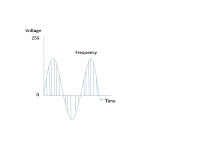## Wednesday, May 20, 2009

### Why Does EPBX Require 64KbpsIn this post, I will be exploring why EPBX requires 64Kbps of bandwidth to deliver end to end voice. To understand the concept, one should know how analog packets converts into digital packets. To convert analog packets into digital, sampling of packets is required. It can be done with the help of Nyquist theorem.
As per Nyquist theorem, sample the signal with double of its frequency to convert it into digital format. Human ear can understand from 20 - 20000 Hz, human can peak between 200 - 9000 Hz. A telephone channel can generate frequency between 300 - 3400 Hz and Nyquist theorem can convert the packets which fall in between 300 - 4000 Hz. If we want to convert the packets which are flowing in telephone channel, we need to twice the frequency. A telephone channel cannot generate more than 3400Hz and its twice will not cross the nyquest theorem. Lets consider if frequency is 4000Hz, double it which is 8000Hz. So we need to create 8000 samples in single second.
1 Sample = 1/8000 Sec

This is pure analog format. To convert it into digital format we need to calculate in terms of numbers and the process is known as quantization. Every second is divided into 8000 number of samples and each sample represents a scale of 256. As we move higher the difference between the number scale increases. It means 8000 samples per seconds and each sample is of 1 byte. Now calcute

8000 samples * 8 bits (1 Bytes) = 64000bps or 64Kbps

This is the bandwidth required for Epbx to set up end to end voice system.

regards
shivlu jain